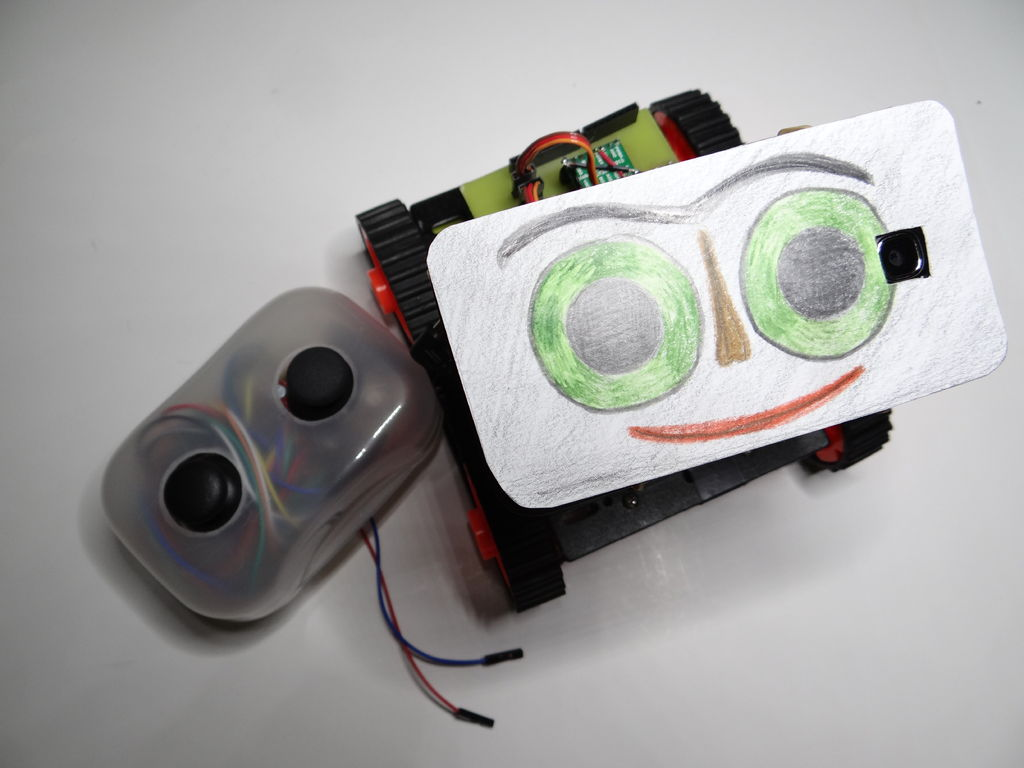I often run out of digital pins on my Arduino. Anything as complicated as, say, a video game controller, was near impossible with the amount of pins I had available. Multiplexing buttons works, but it requires lots of connections and soldering. So, I put together a solution!## Step 1: Resistor Dividers in Five

In a circuit consisting of one resistor and a 9V battery, the resistor drops 9V across itself. So, the resistor’s forward voltage is 9V. Two resistors of equal value would each drop half the voltage, 4.5V, each being half the total resistance in the circuit. If one resistor is 100 ohms, and a second resistor is 300 ohms, each will have a proportional forward voltage. In a 12V circuit, the 100 ohm resistor would drop 3V and the 300 ohm resistor would drop 9V. There’s more to resistor dividers (such as potentiometers), but that is beyond the scope of this instructable. I suggest reading this for more info: https://www.sparkfun.com/tutorials/207

## Step 2: Schematics and Explanation

The first diagram above is the standard way to connect buttons. Each connects to its own digital pin, and each has its own pull-down resistor. In the second diagram, all of the buttons connect to the same analog pin.  They also connect to positive via a 1K ohm resistor, and negative through different value resistors. When button 1 is pressed, the voltage level at Analog 0 is equal to {R2 / (R1+R2)} * Vcc or {100 / (1000+100)} * 5 = 5/11 of a volt or 0.45V. Solving for the other two buttons with 330 and 470 ohm resistors, we get 1.24V for button 2 and about 1.6V for button 3. We could continue adding buttons with higher value resistors, such as 680 ohms, 1.5K ohms, 3.3K ohms, and so on.

READ  Programming an ATtiny w/ Arduino 1.0Also useful is the fact that pressing two buttons simultaneously will produce a completely independent value. Based on the equation for parallel resistors, 1/R=1/R1 +1/R2 … 1/Rx, we can determine that buttons one and two pressed together will produce an overall resistance of 1/100 +1/330 =1/R, so solving for R, the result is about 77 ohms. Plug that into the voltage divider formula with 77 ohms as R2, {77 / (1077)} * 5 = 0.35V, we get an entirely new voltage reading. Possible uses include being able to read diagonals on joysticks that use buttons, like this one: https://www.sparkfun.com/products/9182, without several if..then…else if statements on the Arduino.

### Major Components in Project

Arduino

For more detail: Connect several digital inputs to one analog input using Arduino